Courses

# Test: Air-duct Design

## 10 Questions MCQ Test Topicwise Question Bank for Mechanical Engineering | Test: Air-duct Design

Description
This mock test of Test: Air-duct Design for Mechanical Engineering helps you for every Mechanical Engineering entrance exam. This contains 10 Multiple Choice Questions for Mechanical Engineering Test: Air-duct Design (mcq) to study with solutions a complete question bank. The solved questions answers in this Test: Air-duct Design quiz give you a good mix of easy questions and tough questions. Mechanical Engineering students definitely take this Test: Air-duct Design exercise for a better result in the exam. You can find other Test: Air-duct Design extra questions, long questions & short questions for Mechanical Engineering on EduRev as well by searching above.
QUESTION: 1

### A duct is said to be a low velocity duct if the velocity of air in the duct is upto

Solution:

Low velocity duct, v< 600 m/min

High velocity duct, v > 600 m/min

QUESTION: 2

### Consider the following statements: 1. The duct may be made of galvanised icon, aluminium and fibre glass. 2. The fibre glass may be used in low velocity applications. 3. The pressure in the duct are usually expressed in mm of water 4. High pressure ducts are designed for static pressure upto 150 mm of water. Q. Which of the above statements are valid?

Solution:

Low pressure duct, static pressure < 50 mm of water.
Medium pressure duct, static pressure upto 150 mm of water.
High pressure duct, static pressure vary from 150 to 250 mm of water.

QUESTION: 3

### If the velocity of air flowing duct is V/s, then the velocity pressure (Pv) in mm of water is given by

Solution: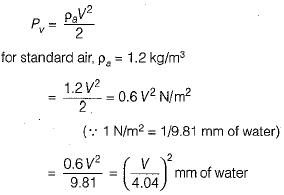QUESTION: 4

When the quality of air passing through the rectangular and circular ducts is same, then the equivalent diameter (D) of a circular duct for a rectangular duct for the same pressure loss per unit length, is given by

Solution:

Pressure loss due to friction,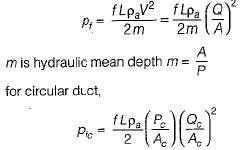for rectangular duc,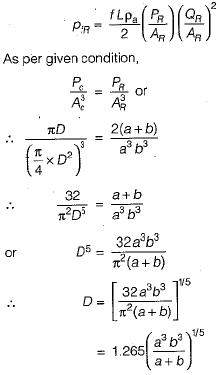QUESTION: 5

For rectangular ducts, the aspect ratio is equal to

Solution:
QUESTION: 6

The static regain method of designing the ducts as compared to equal friction method

Solution:

in static regain method, the size of duct is decided to give equal pressure at all outlets for perfect balancing of the air duct layout system. This method allows for balancing but reducing velocity increases duct size and it should not be taken beyond the economic limit.

QUESTION: 7

When the velocity of air passing through the rectangular and circular ducts is same, then the" equivalent diameter (D) of a circular duct for a rectangular duct for the same pressure loss per unit length, is given by

Solution:

Pressure loss,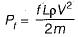for the given condition, mc = mR [Hydraulic mean depth, m]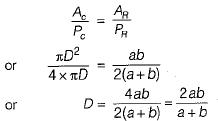QUESTION: 8

The aspect ratio, for rectangular ducts, should not be greater than .... in any case

Solution:

The material required for the rectangular duct increases with aspect ratio.

QUESTION: 9

The main supply air duct of an air-conditioning system is 100 cm x 90 cm in cross-section and carries 10 m3/s of air. It branches off into two ducts, one 80 cm x 80 cm and the other 80 cm x 60 cm. If the mean velocity in the larger branch is 9 m/s, what is the mean velocity in smaller branch?

Solution: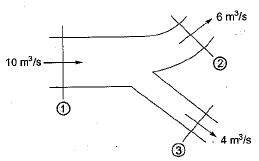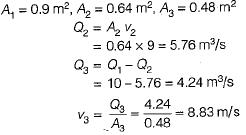QUESTION: 10

Which of the following method(s) is/are adopted in the design of air duct system?
1. Velocity reduction
2. Equal friction
3. Static regain

Q. Select the correct answer using the codes given below:

Solution: